# AP SSC 10th Class Maths Solutions Chapter 1 Real Numbers Ex 1.5

AP State Board Syllabus AP SSC 10th Class Maths Textbook Solutions Chapter 1 Real Numbers Ex 1.5 Textbook Questions and Answers.

## AP State Syllabus SSC 10th Class Maths Solutions 1st Lesson Real Numbers Exercise 1.5

### 10th Class Maths 1st Lesson Real Numbers Ex 1.5 Textbook Questions and Answers

Question 1.
Determine the values of the following,
i) log255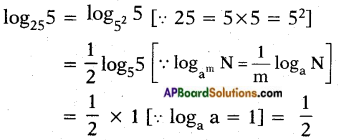ii) log813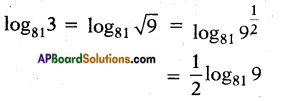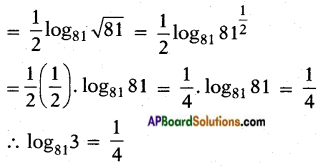iii) log2($$\frac{1}{16}$$)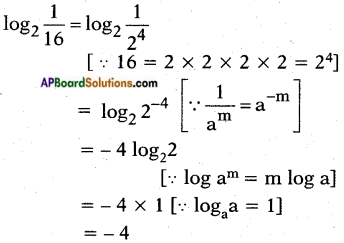iv) log71
log71 = log770 = 0 log77 = 0v) logx√x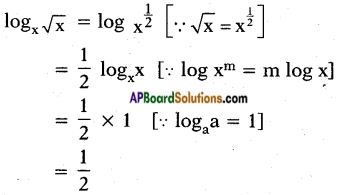vi) log2512
log2512 = log229    [∵ 512 = 29]
= 9log22   [∵ log xm = m log x]
= 9 × 1    [∵ logaa = 1]
= 9

vii) log100.01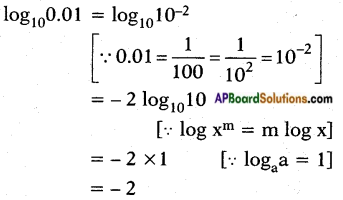viii) $$\log _{\frac{2}{3}}\left(\frac{8}{27}\right)$$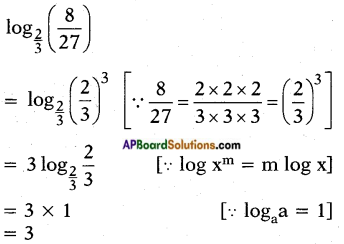ix) $$2^{2+\log _{2} 3}$$
$$2^{2+\log _{2} 3}$$ = 22 . $$2^{\log _{2} 3}$$   [∵ am . an = am+n]
= 4 × 3 [∵ $$\log _{\mathrm{a}} \mathrm{N}$$ = N]
= 12Question 2.
Write the following expressions as log N and find their values.
i) log 2 + log 5
log 2 + log 5
= log 2 × 5   [∵ log m + log n = log mn]
= log 10
= 1

ii) log2 16 – log2 2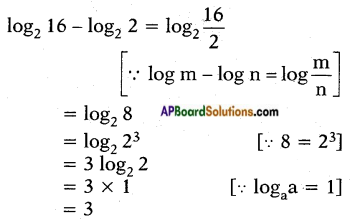iii) 3 log644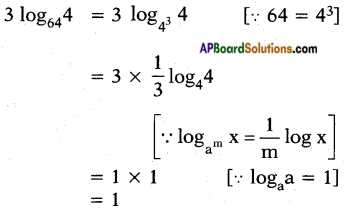iv) 2 log 3 – 3 log 2
2 log 3 – 3 log 2
= log 32 – log 23
= log 9 – log 8
= log $$\frac{9}{8}$$

v) log 10 + 2 log 3 – log 2
log 10 + 2 log 3 – log 2
= log 10 + log 32 – log 2
= log 10 + log 9 – log 2    [∵ m log a = log am]
= log $$\frac{10 \times 9}{2}$$    [∵ log a + log b = log ab; log a – log b = log $$\frac{a}{b}$$]
= log 45Question 3.
Evaluate each of the following in terms of x and y, if it is given x = log23 and y = log2 5.
i) log215
log215 = log2 3 × 5
= log23 + log25   [∵ log mn = log m + log n]
= x + y

ii) log27.5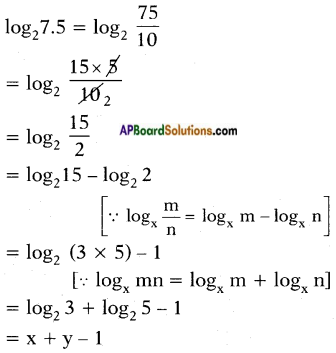iii) log260
log260 = log222 × 3 × 5
= log222 + log23 + log25
= 2 log22 + x + y
= 2 + x + y

iv) log26750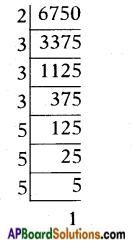log26750
= log22 × 33 × 53
= log22 + log233 + log253
= 1 + 3 log23 + 3 log25
= 1 + 3x + 3yQuestion 4.
Expand the following,
i) log 1000
log 1000 = log 103
= 3 log 10
= 3 × 1
= 3

ii) $$\log \left[\frac{128}{625}\right]$$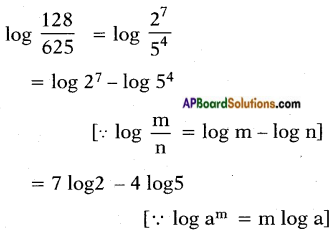iii) log x2y3z4
log x2y3z4 = logx2 + logy3 + logz4 [∵ log ab = log a + log b]
= 2 log x + 3 log y + 4 log z
[∵ log am = m log a]

iv) $$\log \frac{\mathbf{p}^{2} \mathbf{q}^{3}}{\mathbf{r}}$$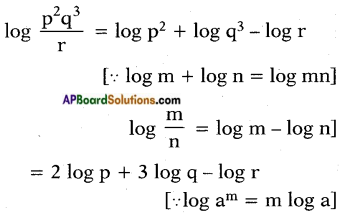iv) $$\log \sqrt{\frac{x^{3}}{y^{2}}}$$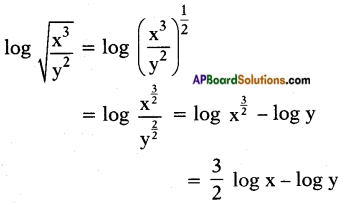Question 5.
If x2 + y2 = 25xy, then prove that 2 log (x + y) = 3log3 + logx + logy.
Given: x2 + y2 = 25xy
We know that (x + y)2 = x2 + y2 + 2xy
= 25xy + 2xy    [∵ x2 + y2 = 25xy given]
(x + y)2 = 27xy
Taking ‘log’ on both sides
log (x + y)2 = log 27xy
2 log (x + y) = log 27 + log x + log y
= log 33 + log x + log y
⇒ 2 log (x + y) = 3log3 + log x + log y

Question 6.
If $$\log \left(\frac{\mathbf{x}+\mathbf{y}}{3}\right)$$ = $$\frac{1}{2}$$ (log x + log y), then find the value of $$\frac{x}{y}+\frac{y}{x}$$.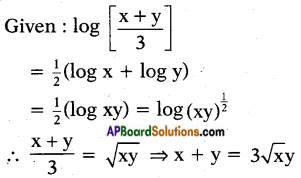(squaring on both sides)
⇒ (x + y)2 = (3√xy)2
⇒ x2 + y2 + 2xy = 9xy
⇒ x2 + y2 = 9xy – 2xy = 7xy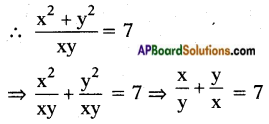Question 7.
If (2.3)x = (0.23)y = 1000 then find the value of $$\frac{1}{x}-\frac{1}{y}$$.
Given (2.3)x = (0.23)y = 1000 = 103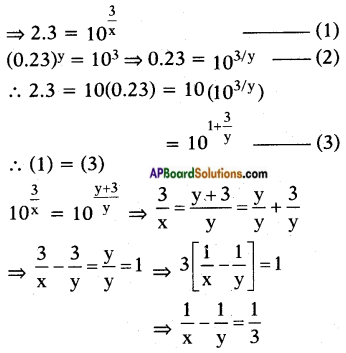Question 8.
If 2x+1 = 31-x then find the value of x.
Given: 2x+1 = 31-x
log 2x+1 = log 31-x
(x + 1) log 2 = (1 – x) log 3
x log 2 + log 2 = log 3 – x log 3
x log 2 + x log 3 = log 3 – log 2
x (log 3 + log 2) = log 3 – log 2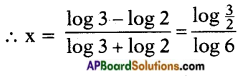Question 9.
Is